# Augmented Matrices and The Gauss-Jordan Method

 Augmented Matrices and The Gauss-Jordan Method (Teacher Notes) Use the information below to set up a system of equations and then solve the system using the elimination method. An investor has a total of 45 one-ounce ingots, made of either gold or silver, worth \$7636.50. The value of a gold ingot is \$280.00, and the value of a silver ingot is \$4.25. Find g, the number of gold ingots, and s, the number of silver ingots? (Released TAKS – July 2006) System of Equations Augmented Matrices Answer: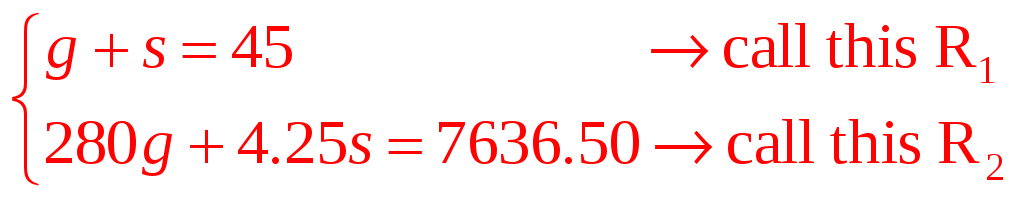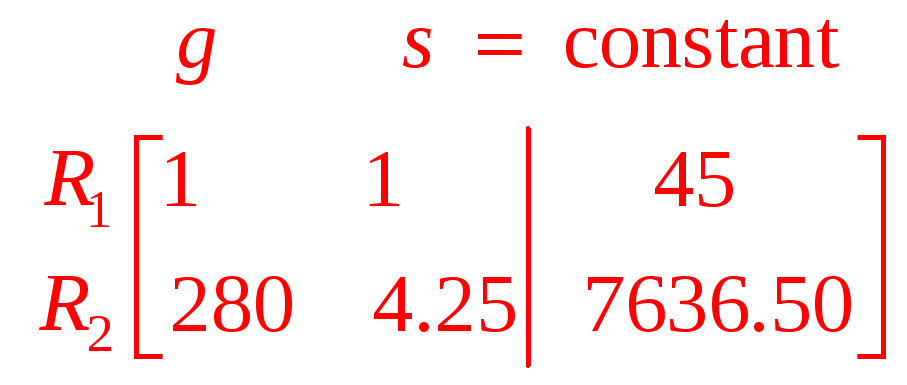1st : Find s by eliminating g.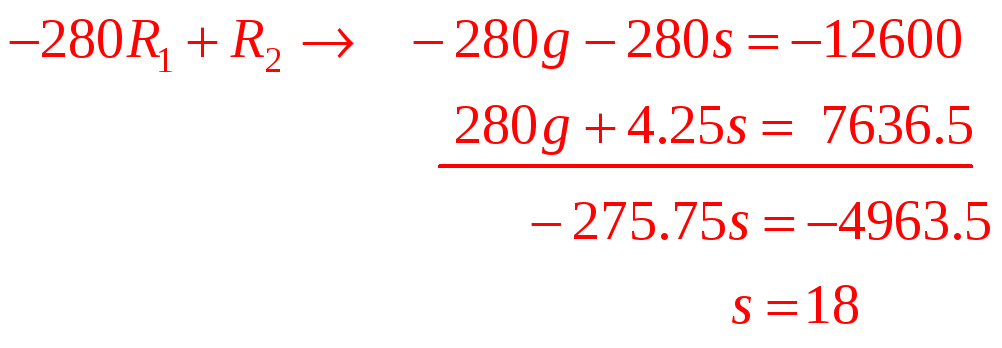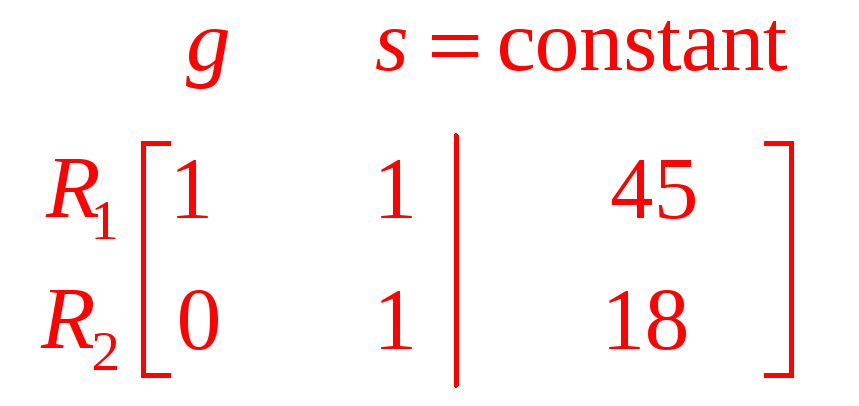2nd : Find g by eliminating s in R1.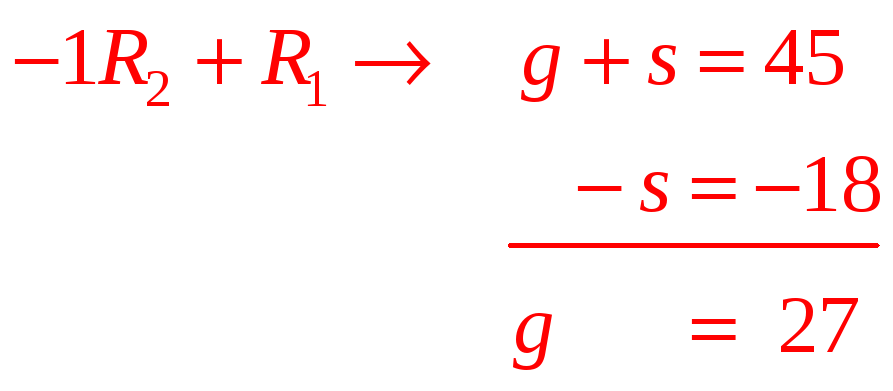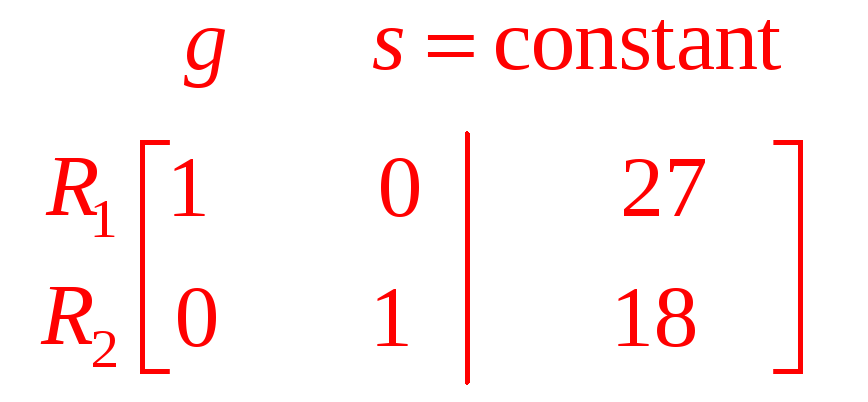The process used above is called The Gauss-Jordan Elimination Method. This is a systematic way to solve system of equations and is especially helpful when solving very large systems of equations. The first step in using the Gauss-Jordan Elimination Method is to use the system of equations to set up an augmented matrix (a coefficient matrix next to a constant matrix) . For the problems below, set up a system of equations and the corresponding augmented matrix. Download 0.67 Mb.Share with your friends: